# What is Image Compression?

In the field of Image processing, the compression of images is an important step before we start the processing of larger images or videos. The compression of images is carried out by an encoder and output a compressed form of an image. In the processes of compression, the mathematical transforms play a vital role. A flow chart of the process of the compression of the image can be represented as: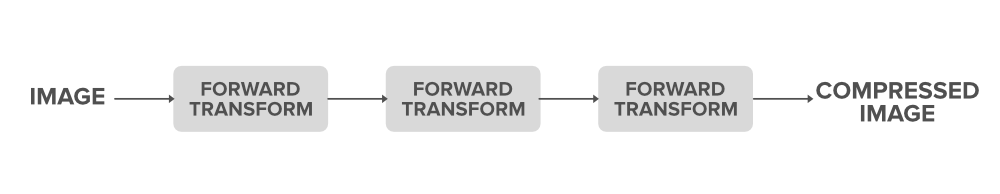In this article, we try to explain the overview of the concepts involved in the image compression techniques. The general representation of the image in a computer is like a vector of pixels. Each pixel is represented by a fixed number of bits. These bits determine the intensity of the color (on greyscale if a black and white image and has three channels of RGB if colored images.)

### Why Do We Need Image Compression?

Consider a black and white image that has a resolution of 1000*1000 and each pixel uses 8 bits to represent the intensity. So the total no of bits req= 1000*1000*8 = 80,00,000 bits per image. And consider if it is a video with 30 frames per second of the above-mentioned type images then the total bits for a video of 3 secs is: 3*(30*(8, 000, 000))=720, 000, 000 bits

As we see just to store a 3-sec video we need so many bits which is very huge. So, we need a way to have proper representation as well to store the information about the image in a minimum no of bits without losing the character of the image. Thus, image compression plays an important role.

Basic steps in image compression:

• Applying the image transform
• Quantization of the levels
• Encoding the sequences.

### Transforming The Image

What is a transformation(Mathematically):

It a function that maps from one domain(vector space) to another domain(other vector space). Assume, T is a transform, f(t):X->X’ is a function then, T(f(t)) is called the transform of the function.

In a simple sense, we can say that T changes the shape(representation) of the function as it is a mapping from one vector space to another (without changing basic function f(t) i.e the relationship between the domain and co-domain).

We generally carry out the transformation of the function from one vector space to the other because when we do that in the newly projected vector space we infer more information about the function.

A real life example of a transform:Here we can say that the prism is a transformation function in which it splits the white light (f(t)) into its components i.e the representation of the white light.
And we observe that we can infer more information about the light in its component representation than the white light one. This is how transforms help in understanding the functions in an efficient manner.

### Transforms in Image Processing

The image is also a function of the location of the pixels. i.e I(x, y) where (x, y) are the coordinates of the pixel in the image. So we generally transform an image from the spatial domain to the frequency domain.

Why Transformation of the Image is Important:

• It becomes easy to know what all the principal components that make up the image and help in the compressed representation.
• It makes the computations easy.
• Example: finding convolution in the time domain before the transformation: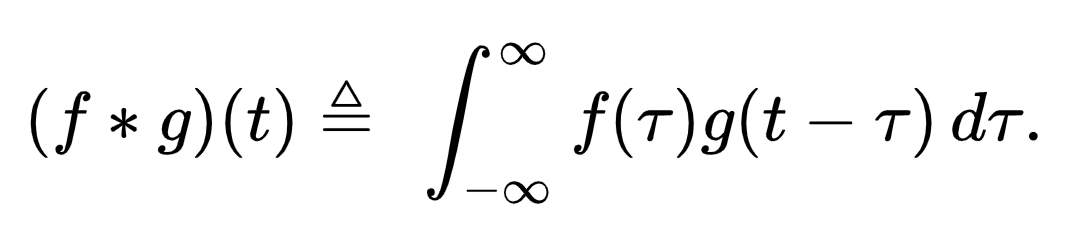Finding convolution in the frequency domain after the transformation: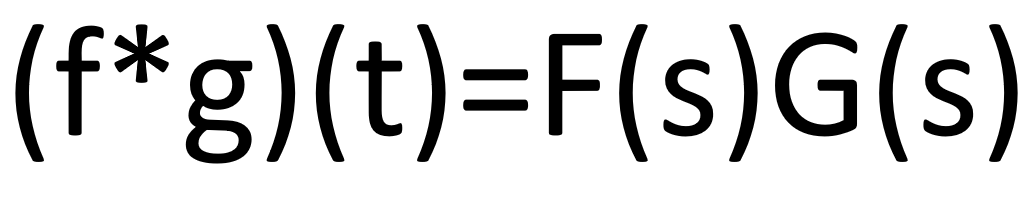• So we can see that the computation cost has reduced as we switched to the frequency domain. We can also see that in the time domain the convolution was equivalent to an integration operator but in the frequency domain, it becomes equal to the simple product of terms. So, this way the cost of computation reduces.

So this way when we transform the image from domain to the other carrying out the spatial filtering operations becomes easier.

### Quantization

The process quantization is a vital step in which the various levels of intensity are grouped into a particular level based on the mathematical function defined on the pixels. Generally, the newer level is determined by taking a fixed filter size of “m” and dividing each of the “m” terms of the filter and rounding it its closest integer and again multiplying with “m”.

#### Basic quantization Function: [pixelvalue/m] * m

So, the closest of the pixel values approximate to a single level hence as the no of distinct levels involved in the image becomes less. Hence we reduce the redundancy in the level of the intensity. So thus quantization helps in reducing the distinct levels.

Eg: (m=9)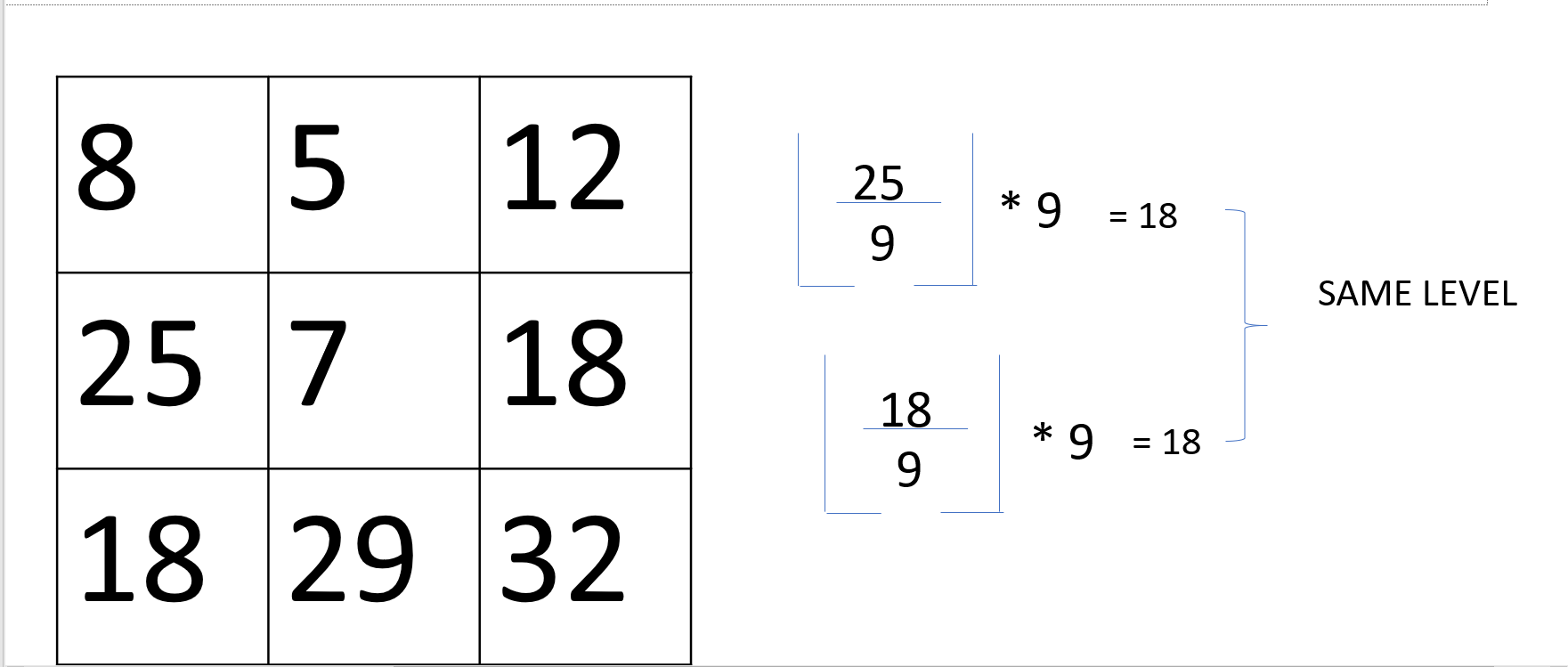Thus we see in the above example both the intensity values round up to 18 thus we reduce the number of distinct levels(characters involved) in the image specification.

### Symbol Encoding

The symbol stage involves where the distinct characters involved in the image are encoded in a way that the no. of bits required to represent a character is optimal based on the frequency of the character’s occurrence. In simple terms, In this stage codewords are generated for the different characters present. By doing so we aim to reduce the no. of bits required to represent the intensity levels and represent them in an optimum number of bits.

There are many encoding algorithms. Some of the popular ones are:

• Huffman variable-length encoding.
• Run-length encoding.

In the Huffman coding scheme, we try to find the codes in such a way that none of the codes are the prefixes to the other. And based on the probability of the occurrence of the character the length of the code is determined. In order to have an optimum solution the most probable character has the smallest length code.

Example: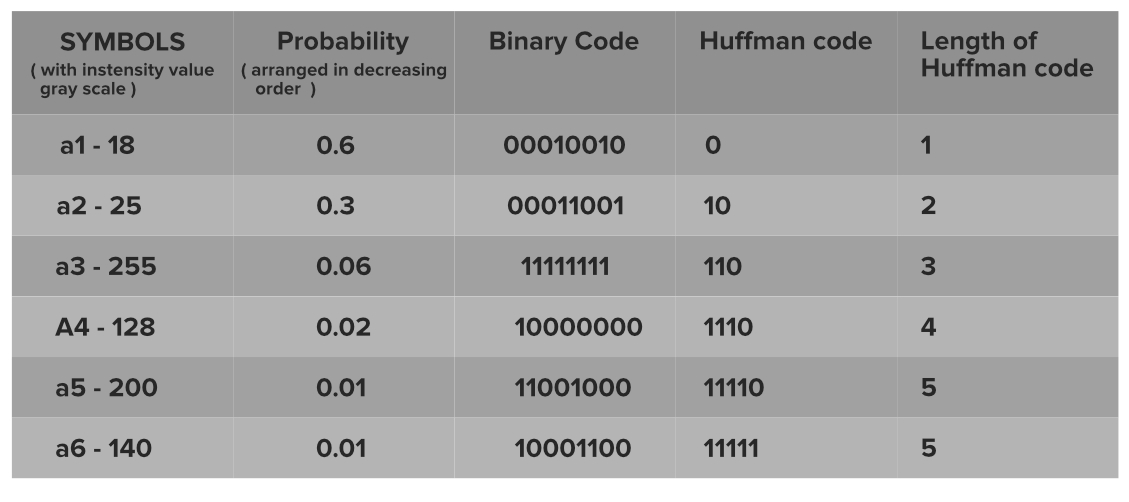We see the actual 8-bit representation as well as the new smaller length codes. The mechanism of generation of codes is: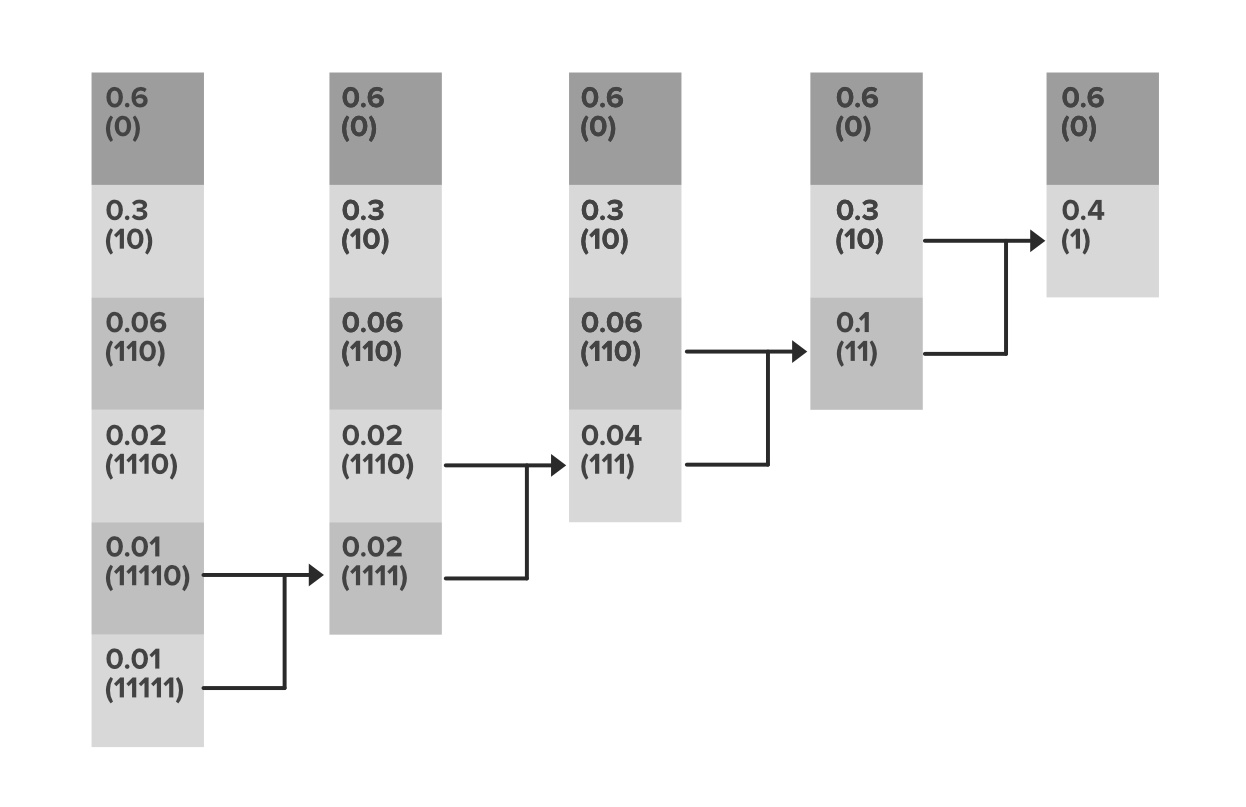So we see how the storage requirement for the no of bits is decreased as:

Initial representation–average code length: 8 bits per intensity level.

After encoding–average code length: (0.6*1)+(0.3*2)+(0.06*3)+(0.02*4)+(0.01*5)+(0.01*5)=1.56 bits per intensity level

Thus the no of bits required to represent the pixel intensity is drastically reduced.

Thus in this way, the mechanism of quantization helps in compression. When the images are once compressed its easy for them to be stored on a device or to transfer them. And based on the type of transforms used, type of quantization, and the encoding scheme the decoders are designed based on the reversed logic of the compression so that the original image can be re-built based on the data obtained out of the compressed images

Attention reader! Don’t stop learning now. Get hold of all the important CS Theory concepts for SDE interviews with the CS Theory Course at a student-friendly price and become industry ready.

My Personal Notes arrow_drop_upCheck out this Author's contributed articles.

If you like GeeksforGeeks and would like to contribute, you can also write an article using contribute.geeksforgeeks.org or mail your article to contribute@geeksforgeeks.org. See your article appearing on the GeeksforGeeks main page and help other Geeks.

Please Improve this article if you find anything incorrect by clicking on the "Improve Article" button below.

Article Tags :

Be the First to upvote.

Please write to us at contribute@geeksforgeeks.org to report any issue with the above content.